# 3.5: Subtraction of Signed Numbers

$$\newcommand{\vecs}{\overset { \rightharpoonup} {\mathbf{#1}} }$$ $$\newcommand{\vecd}{\overset{-\!-\!\rightharpoonup}{\vphantom{a}\smash {#1}}}$$$$\newcommand{\id}{\mathrm{id}}$$ $$\newcommand{\Span}{\mathrm{span}}$$ $$\newcommand{\kernel}{\mathrm{null}\,}$$ $$\newcommand{\range}{\mathrm{range}\,}$$ $$\newcommand{\RealPart}{\mathrm{Re}}$$ $$\newcommand{\ImaginaryPart}{\mathrm{Im}}$$ $$\newcommand{\Argument}{\mathrm{Arg}}$$ $$\newcommand{\norm}{\| #1 \|}$$ $$\newcommand{\inner}{\langle #1, #2 \rangle}$$ $$\newcommand{\Span}{\mathrm{span}}$$ $$\newcommand{\id}{\mathrm{id}}$$ $$\newcommand{\Span}{\mathrm{span}}$$ $$\newcommand{\kernel}{\mathrm{null}\,}$$ $$\newcommand{\range}{\mathrm{range}\,}$$ $$\newcommand{\RealPart}{\mathrm{Re}}$$ $$\newcommand{\ImaginaryPart}{\mathrm{Im}}$$ $$\newcommand{\Argument}{\mathrm{Arg}}$$ $$\newcommand{\norm}{\| #1 \|}$$ $$\newcommand{\inner}{\langle #1, #2 \rangle}$$ $$\newcommand{\Span}{\mathrm{span}}$$$$\newcommand{\AA}{\unicode[.8,0]{x212B}}$$

## Overview

• Definition of Subtraction
• Subtraction of Signed Numbers

## Definition of Subtraction

We know from our experience with arithmetic that the subtraction $$5−2$$ produces $$3$$, that is, $$5−2=3$$. Illustrating this process on the number line suggests a rule for subtracting signed numbers.We begin at $$0$$, the origin.
Since $$5$$ is positive, we move $$5$$ units to the right.
Then, we move 2 units to the left to get to $$3$$. (This reminds us of addition with a negative number.)

This illustration suggests that $$5−2$$ is the same as $$5+(−2)$$.
This leads us directly to the definition of subtraction.

##### Definition of Subtraction

If $$a$$ and $$b$$ are real numbers, $$a−b$$ is the same as $$a+(−b)$$, where $$−b$$ is the opposite of $$b$$.

## Subtraction of Signed Numbers

The preceding definition suggests the rule for subtracting signed numbers.

##### Subtraction of Signed Numbers

To perform the subtraction $$a−b$$, add the opposite of $$b$$ to $$a$$, that is, change the sign of $$b$$ and add.

## Sample Set A

Perform the subtractions.

##### Example $$\PageIndex{1}$$

$$5−3=5+(−3)=2$$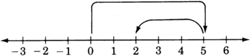##### Example $$\PageIndex{2}$$

$$4−9=4+(−9)=−5$$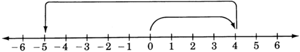##### Example $$\PageIndex{3}$$

$$−4−6=−4+(−6)=−10$$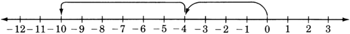##### Example $$\PageIndex{4}$$

$$−3−(−12)=−3+12=9$$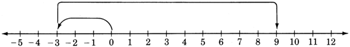##### Example $$\PageIndex{5}$$

$$0−(−15)=0+15=15$$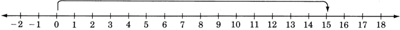##### Example $$\PageIndex{6}$$

The high temperature today in Lake Tahoe was 26F. The low temperature tonight is expected to be −7F. How many degrees is the temperature expected to drop?
We need to find the difference between 26 and −7.

$$26−(−7)=26+7=33$$

Thus, the expected temperature drop is 33F.

##### Example $$\PageIndex{7}$$

\begin{aligned} -6-(-5)-10 &=-6+5+(-10) \\ &=(-6+5)+(-10) \\ &=-1+(-10) \\ &=-11 \end{aligned}

## Practice Set A

Perform the subtractions.

##### Practice Problem $$\PageIndex{1}$$

$$9−6$$

$$3$$

##### Practice Problem $$\PageIndex{2}$$

$$6-9$$

$$-3$$

##### Practice Problem $$\PageIndex{3}$$

$$0-7$$

$$-7$$

##### Practice Problem $$\PageIndex{4}$$

$$1-14$$

$$-13$$

##### Practice Problem $$\PageIndex{5}$$

$$-8-12$$

$$-20$$

##### Practice Problem $$\PageIndex{6}$$

$$-21-6$$

$$-27$$

##### Practice Problem $$\PageIndex{7}$$

$$-6-(-4)$$

$$-2$$

##### Practice Problem $$\PageIndex{8}$$

$$8-(-10)$$

$$18$$

##### Practice Problem $$\PageIndex{9}$$

$$1-(-12)$$

$$13$$

##### Practice Problem $$\PageIndex{10}$$

$$86-(-32)$$

$$118$$

##### Practice Problem $$\PageIndex{11}$$

$$0-16$$

$$-16$$

##### Practice Problem $$\PageIndex{12}$$

$$0-(-16)$$

$$16$$

##### Practice Problem $$\PageIndex{13}$$

$$0-(8)$$

$$-8$$

##### Practice Problem $$\PageIndex{14}$$

$$5-(-5)$$

$$10$$

##### Practice Problem $$\PageIndex{15}$$

$$24-(-(-24))$$

$$0$$

## Exercises

For the following exercises, perform the indicated operations.

##### Exercise $$\PageIndex{1}$$

$$8-3$$

$$5$$

##### Exercise $$\PageIndex{2}$$

$$12 - 7$$

$$5$$

##### Exercise $$\PageIndex{3}$$

$$5-6$$

$$-1$$

##### Exercise $$\PageIndex{4}$$

$$14 - 30$$

##### Exercise $$\PageIndex{5}$$

$$2 - 15$$

$$-13$$

##### Exercise $$\PageIndex{6}$$

$$5 - 18$$

$$-13$$

##### Exercise $$\PageIndex{7}$$

$$1 - 7$$

$$-6$$

##### Exercise $$\PageIndex{8}$$

$$4 - 11$$

##### Exercise $$\PageIndex{9}$$

$$-6 - 5$$

$$-11$$

##### Exercise $$\PageIndex{10}$$

$$-8 - 14$$

##### Exercise $$\PageIndex{11}$$

$$-1 - 12$$

$$-13$$

##### Exercise $$\PageIndex{12}$$

$$-4 - 4$$

##### Exercise $$\PageIndex{13}$$

$$-6 - 8$$

$$-14$$

##### Exercise $$\PageIndex{14}$$

$$-1 - 12$$

$$-13$$

##### Exercise $$\PageIndex{15}$$

$$-5 - (-3)$$

$$-2$$

##### Exercise $$\PageIndex{16}$$

$$-11 - (-8)$$

##### Exercise $$\PageIndex{17}$$

$$-7 -(-12)$$

$$5$$

##### Exercise $$\PageIndex{18}$$

$$-2 -(-10)$$

##### Exercise $$\PageIndex{19}$$

$$-4 -(-15)$$

$$11$$

##### Exercise $$\PageIndex{20}$$

$$-11 -(-16)$$

##### Exercise $$\PageIndex{21}$$

$$-1 -(-6)$$

$$5$$

##### Exercise $$\PageIndex{22}$$

$$-8 -(-14)$$

##### Exercise $$\PageIndex{23}$$

$$-15 -(-10)$$

$$-5$$

##### Exercise $$\PageIndex{24}$$

$$-11 -(-4)$$

##### Exercise $$\PageIndex{25}$$

$$-16 -(-8)$$

$$-8$$

##### Exercise $$\PageIndex{26}$$

$$-12 -(-11)$$

##### Exercise $$\PageIndex{27}$$

$$0 - 6$$

$$-6$$

##### Exercise $$\PageIndex{28}$$

$$0 - 15$$

##### Exercise $$\PageIndex{29}$$

$$0 - (-7)$$

$$7$$

##### Exercise $$\PageIndex{30}$$

$$0 - (-10)$$

##### Exercise $$\PageIndex{31}$$

$$67 - 38$$

$$29$$

##### Exercise $$\PageIndex{32}$$

$$142 - 85$$

##### Exercise $$\PageIndex{33}$$

$$816 - 1140$$

$$-324$$

##### Exercise $$\PageIndex{34}$$

$$105-421$$

##### Exercise $$\PageIndex{35}$$

$$-550 - (-121)$$

$$-429$$

##### Exercise $$\PageIndex{36}$$

$$−15.016−(4.001)$$

##### Exercise $$\PageIndex{37}$$

$$−26+7−52$$

$$-71$$

##### Exercise $$\PageIndex{38}$$

$$−15−21−(−2)$$

##### Exercise $$\PageIndex{39}$$

$$−104−(−216)−(−52)$$

$$164$$

##### Exercise $$\PageIndex{40}$$

$$−0.012−(−0.111)−(0.035)$$

##### Exercise $$\PageIndex{41}$$

$$[5+(−6)]−[2+(−4)]$$

$$1$$

##### Exercise $$\PageIndex{42}$$

$$[2+(−8)]−[5+(−7)]$$

##### Exercise $$\PageIndex{43}$$

$$[4+(−11)]−[2+(−10)]$$

$$1$$

##### Exercise $$\PageIndex{44}$$

$$[9+(−6)]−[4+(−12)]$$

##### Exercise $$\PageIndex{45}$$

$$(11−8)−(1−6)$$

$$8$$

##### Exercise $$\PageIndex{46}$$

$$(5−12)−(4−10)$$

##### Exercise $$\PageIndex{47}$$

$$(1−10)−(2−15)$$

$$4$$

##### Exercise $$\PageIndex{48}$$

$$(0−8)−(4−12)$$

##### Exercise $$\PageIndex{49}$$

$$(−4+7)−(2−5)$$

$$6$$

##### Exercise $$\PageIndex{50}$$

$$(−6+2)−(5−11)$$

##### Exercise $$\PageIndex{51}$$

$$[−8+(−5+3)]−[9−(−3−5)]$$

$$-27$$

##### Exercise $$\PageIndex{52}$$

$$[−4+(−1+6)]−[7−(−6−1)]$$

##### Exercise $$\PageIndex{53}$$

$$[2−(−6+10)]−[1−(2−11)]$$

$$-12$$

##### Exercise $$\PageIndex{54}$$

$$[5−(−2−5)]−[2−(−1−4)]$$

##### Exercise $$\PageIndex{55}$$

When a particular machine is operating properly, its meter will read 34. If a broken bearing in the machine causes the meter reading to drop by 45 units, what is the meter reading?

$$-11$$

##### Exercise $$\PageIndex{56}$$

The low temperature today in Denver was −4F and the high was 42F. What is the temperature difference?

## Exercises for Review

##### Exercise $$\PageIndex{57}$$

Use the distributive property to expand $$4x(5y+11)$$

$$20xy + 44x$$

##### Exercise $$\PageIndex{58}$$

Simplify $$\dfrac{2(3x^2y^2)^3(2x^4y^3)^0}{27x^4y^3}$$. Assume $$x \not = 0$$, $$y \not = 0$$

##### Exercise $$\PageIndex{59}$$

Simplify $$|-(4^2+2^2-3^2)|$$.

$$11$$

##### Exercise $$\PageIndex{60}$$

Find the sum. $$-8 + (-14)$$

##### Exercise $$\PageIndex{61}$$

Find the sum. $$3 + (-6)$$.

$$-3$$